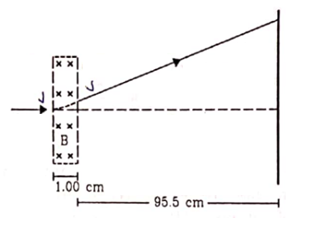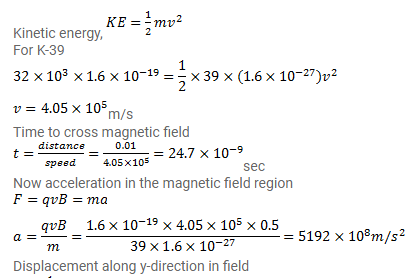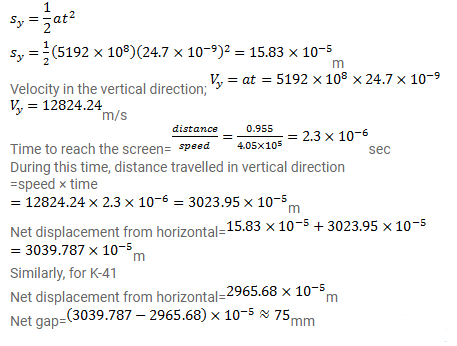# A narrow beam of singly-charged potassium ions of kinetic energy

Question:

A narrow beam of singly-charged potassium ions of kinetic energy $32 \mathrm{keV}$ is injected into a region of width $1.00 \mathrm{~cm}$ having a magnetic field of strength $0.500 T$ as shown in figure. The ions are collected at a screen $95.5 \mathrm{~cm}$ away from the field region. If the beam contains isotopes of atomic weights 39 and 41 , find the separation between the points where these isotopes strike the screen. Take the mass of a potassium ion $=A\left(1.6 \times 10^{-27}\right) \mathrm{kg}$ where A is the mass number.Solution: